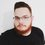# In dividing a equilateral triangle into n equal parts with equal areas and equal shapes...

People of Brilliant, how are you all? I came across an interesting puzzle that I want some help solving.

Suppose we want to divide an equilateral triangle into $n$ equal pieces, with the same area and the same shape. Is it possible to divide such a triangle into $5$ equal pieces? Is it possible to do it for an arbitrary value of $n$?

My investigations showed me that 2 and 3 are the most basic divisions available, and then onward I could find ways to divide a triangle into $n^2$ pieces, and then either into $2n^2, 3n^2$ and $6n^2$. Any hints as to how I can either do it, or prove there is no way?Note by Alexandre Miquilino
3 years, 8 months ago

This discussion board is a place to discuss our Daily Challenges and the math and science related to those challenges. Explanations are more than just a solution — they should explain the steps and thinking strategies that you used to obtain the solution. Comments should further the discussion of math and science.

When posting on Brilliant:

• Use the emojis to react to an explanation, whether you're congratulating a job well done , or just really confused .
• Ask specific questions about the challenge or the steps in somebody's explanation. Well-posed questions can add a lot to the discussion, but posting "I don't understand!" doesn't help anyone.
• Try to contribute something new to the discussion, whether it is an extension, generalization or other idea related to the challenge.

MarkdownAppears as
*italics* or _italics_ italics
**bold** or __bold__ bold
- bulleted- list
• bulleted
• list
1. numbered2. list
1. numbered
2. list
Note: you must add a full line of space before and after lists for them to show up correctly
paragraph 1paragraph 2

paragraph 1

paragraph 2

[example link](https://brilliant.org)example link
> This is a quote
This is a quote
    # I indented these lines
# 4 spaces, and now they show
# up as a code block.

print "hello world"
# I indented these lines
# 4 spaces, and now they show
# up as a code block.

print "hello world"
MathAppears as
Remember to wrap math in $$ ... $$ or $ ... $ to ensure proper formatting.
2 \times 3 $2 \times 3$
2^{34} $2^{34}$
a_{i-1} $a_{i-1}$
\frac{2}{3} $\frac{2}{3}$
\sqrt{2} $\sqrt{2}$
\sum_{i=1}^3 $\sum_{i=1}^3$
\sin \theta $\sin \theta$
\boxed{123} $\boxed{123}$

Sort by:

If the pieces needn't be contiguous, it turns out it can be done as shown here !!

- 3 years, 7 months ago

While this is not exactly a solution I wanted, there is a reference to a paper in another answer which seems to state it is impossible. Thanks for the find!

- 3 years, 7 months ago

Hi,may I ask that where do you live,which country?

- 3 years, 6 months ago

4 join all the midpoints of the sides forming 4 equilateral triangles each of equal area

- 3 years, 5 months ago

4 falls in the $n^2$ category, but thanks for the input.

- 3 years, 5 months ago

oh sorry

- 3 years, 4 months ago